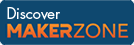Cody

# Problem 44307. The glass half full

Created by HH in Community

Identical glasses are placed in a triangular tower structure, such that the top level (L = 1) comprises one glass, the next level down (L = 2) comprises three glasses, the next level down (L = 3) comprises six glasses, and so on.

Follow the link to see a diagram shows the first three levels. The glasses in each levels are represented by the blue circles, while the yellow circles represent the positions of the glasses in the next higher level.

Water is poured into the top glass at a constant volumetric flow rate. When the glass is filled, the water starts spilling over and into the glasses below. Note that water only spills outward , meaning that at some point, some glasses will remain empty.

Given the volume of a glass in liters, v, the volumetric flow rate in liters per second, u, and an integer, L, representing a level in the glass structure, return the total number of glasses in that level, g, the number of glasses in that level that will be filled with water, f, and the time, in seconds, it would take to fill all "fillable" glasses in that level, t, starting with no water in any of the levels.

Example:

Input: v = 0.25, u = 0.1, L = 2

Output: g = 3, f = 3, t = 10

### Solution Stats

48.23% Correct | 51.77% Incorrect
Last solution submitted on Mar 20, 2019

#### TagsMATLAB and Simulink resources for Arduino, LEGO, and Raspberry Pi